# RS Aggarwal Solutions for Class 6 Chapter 3 Whole Numbers Exercise 3D

RS Aggarwal Solutions for Class 6, Chapter 3, Whole Numbers Exercise 3D are given here with step by step explanation for free download. This exercise has questions about properties of the multiplication of Whole Numbers with the illustrative example for further understanding. These solutions come handy for quickly completing students’ homework. RS Aggarwal Solutions for Class 6 are 100 percent accurate for students who aspire to score high marks in the examinations.

## Download PDF of RS Aggarwal Solutions for Class 6 Chapter 3 Whole Numbers Exercise 3D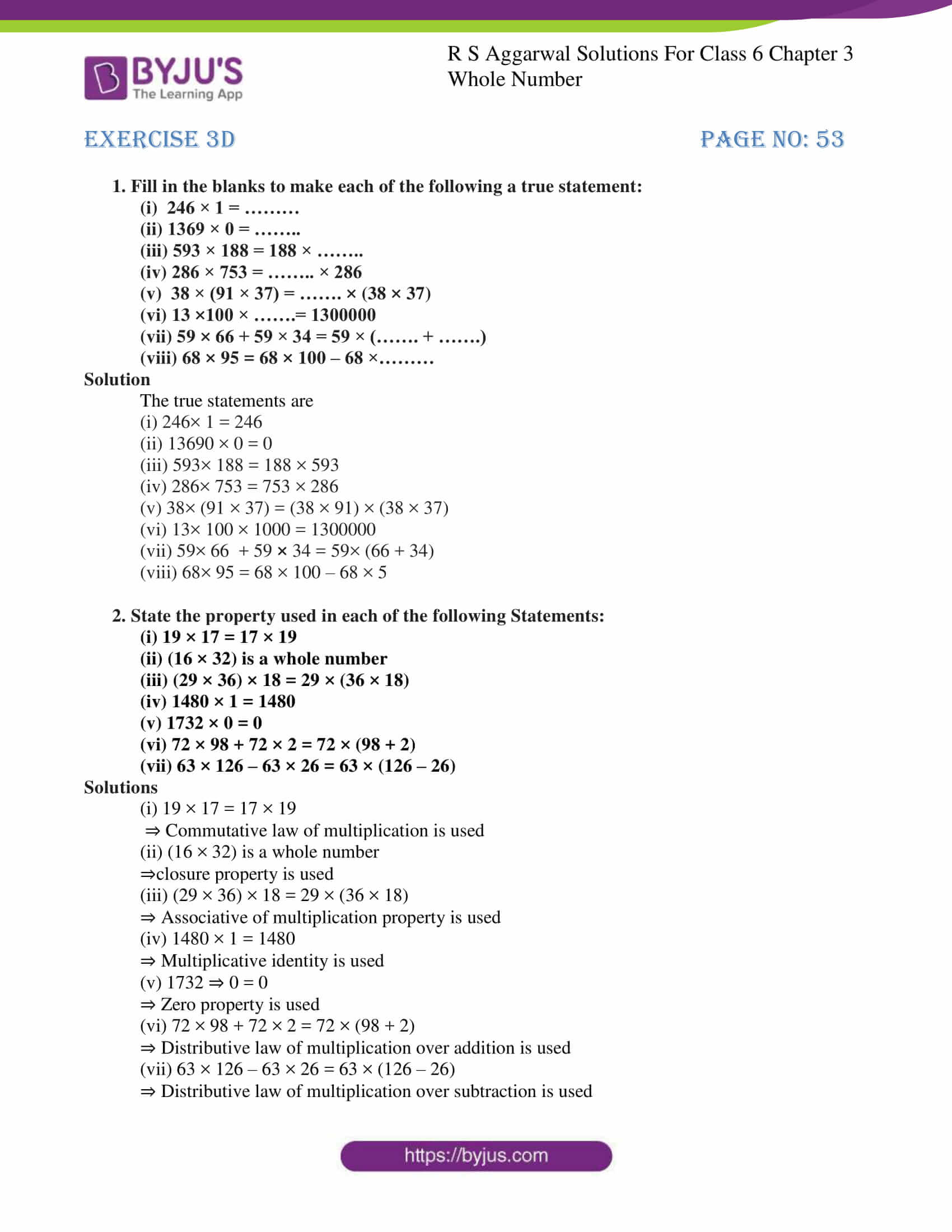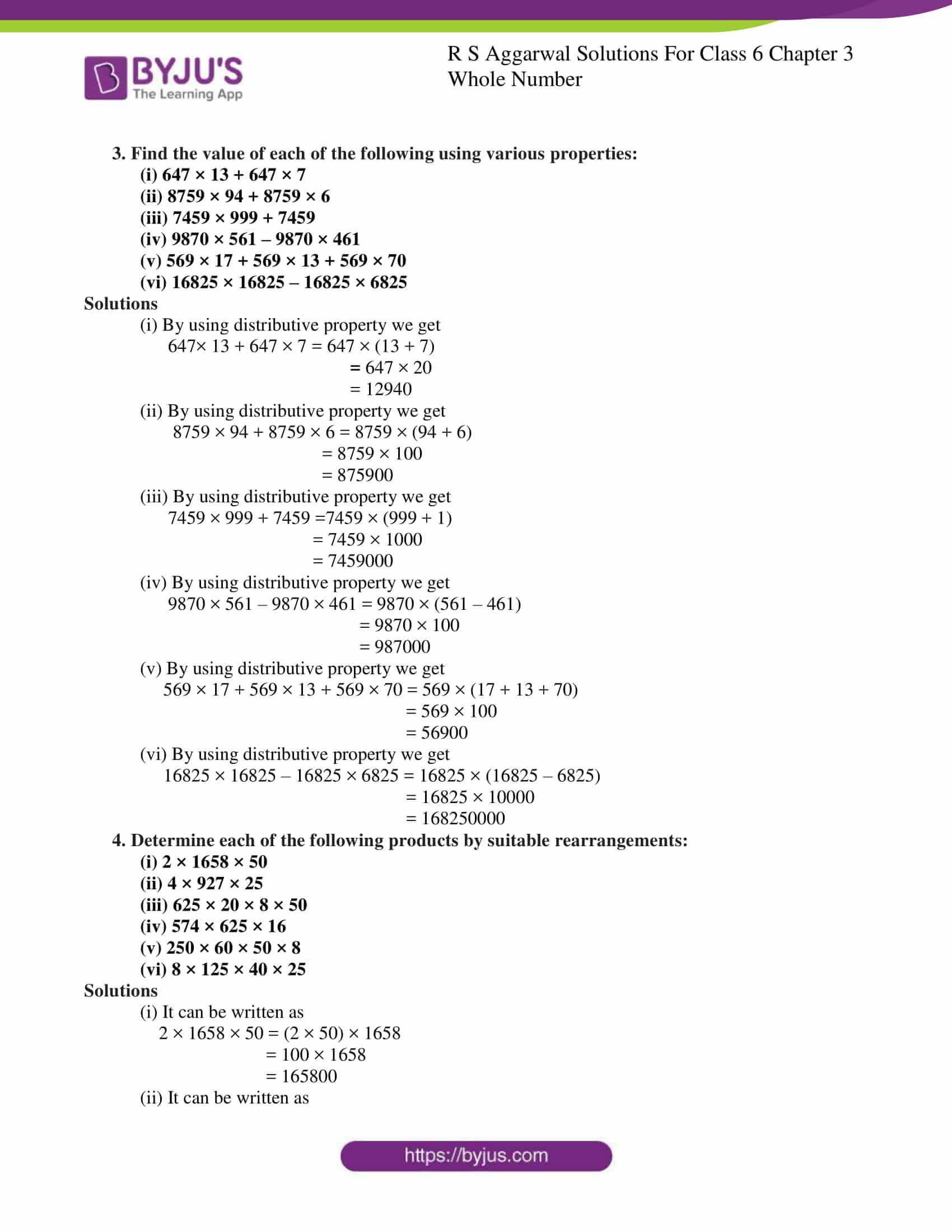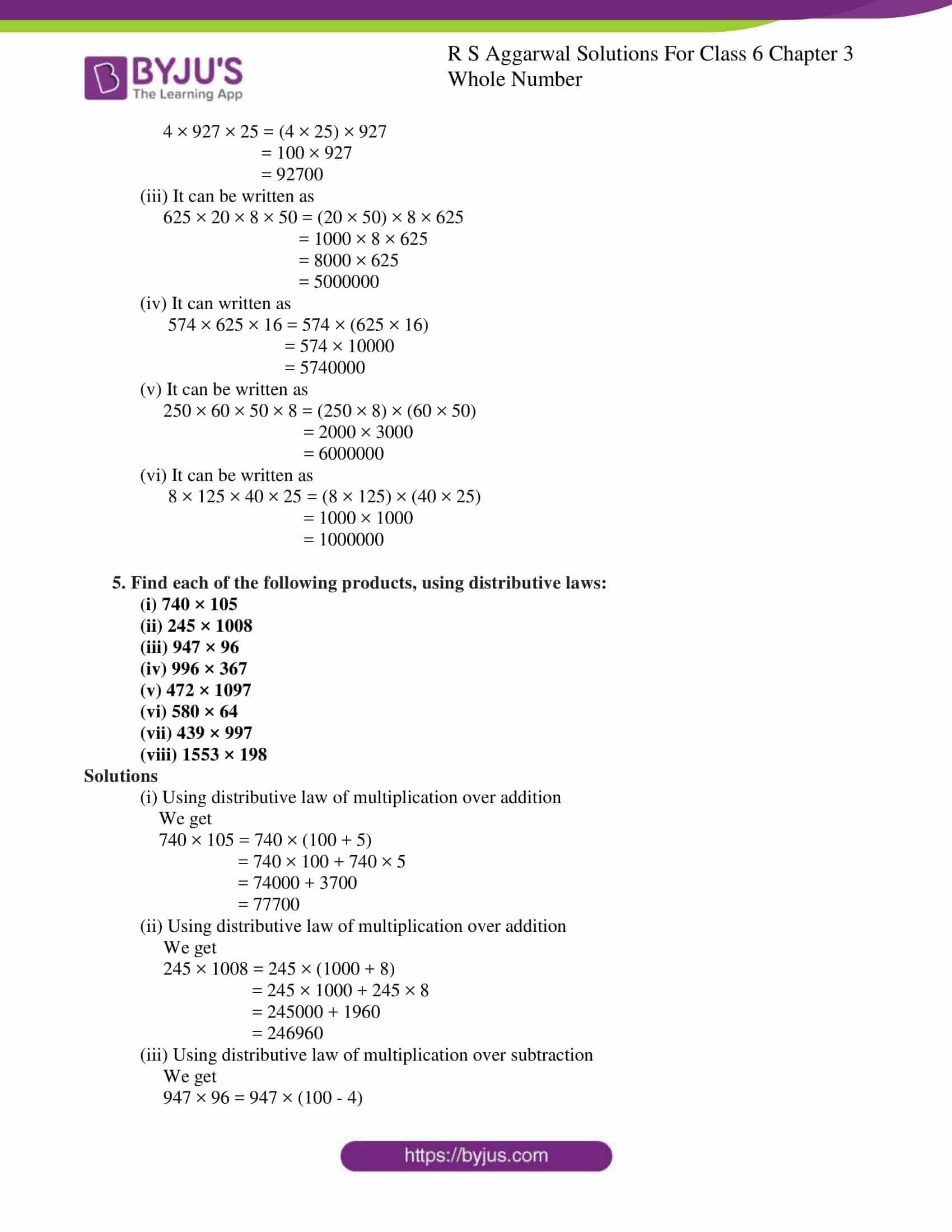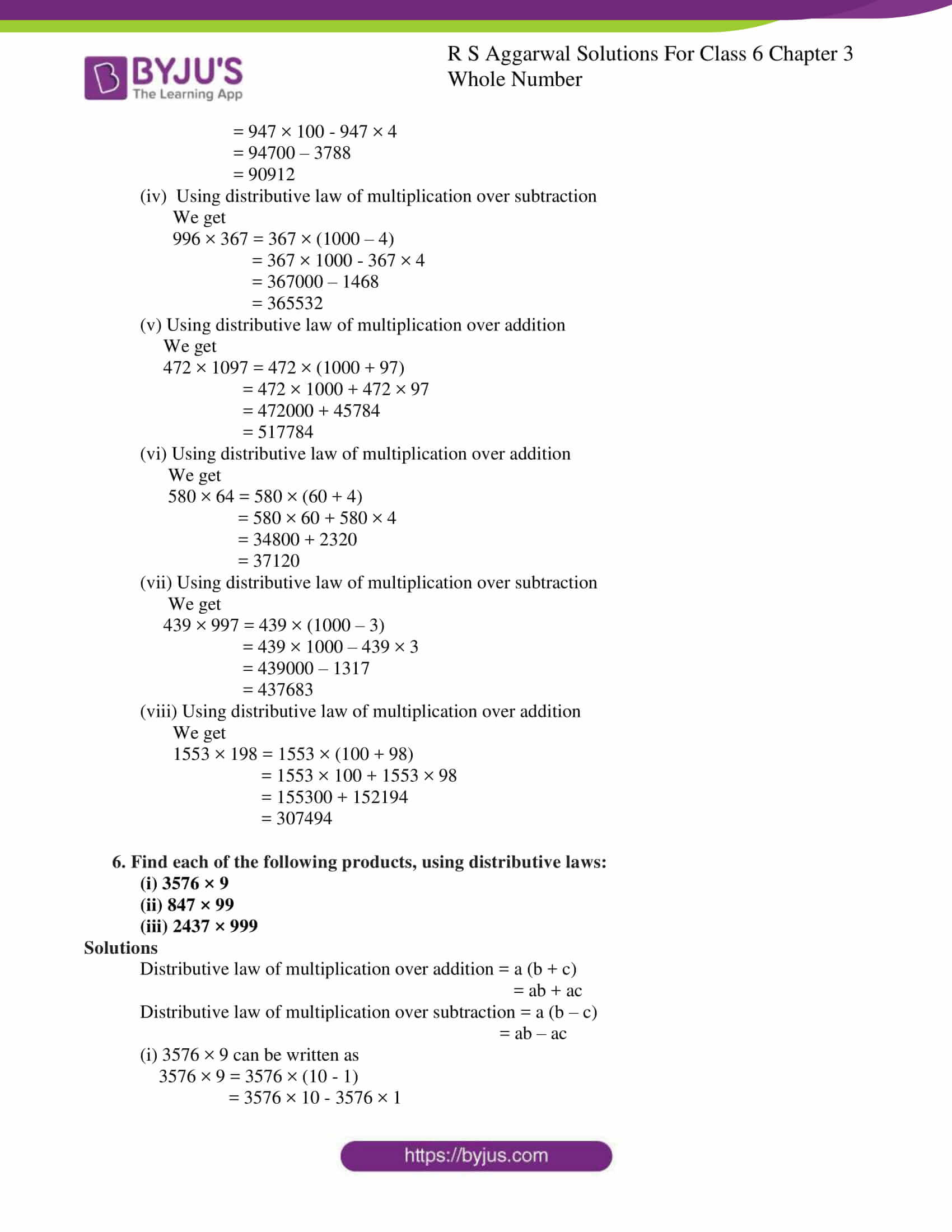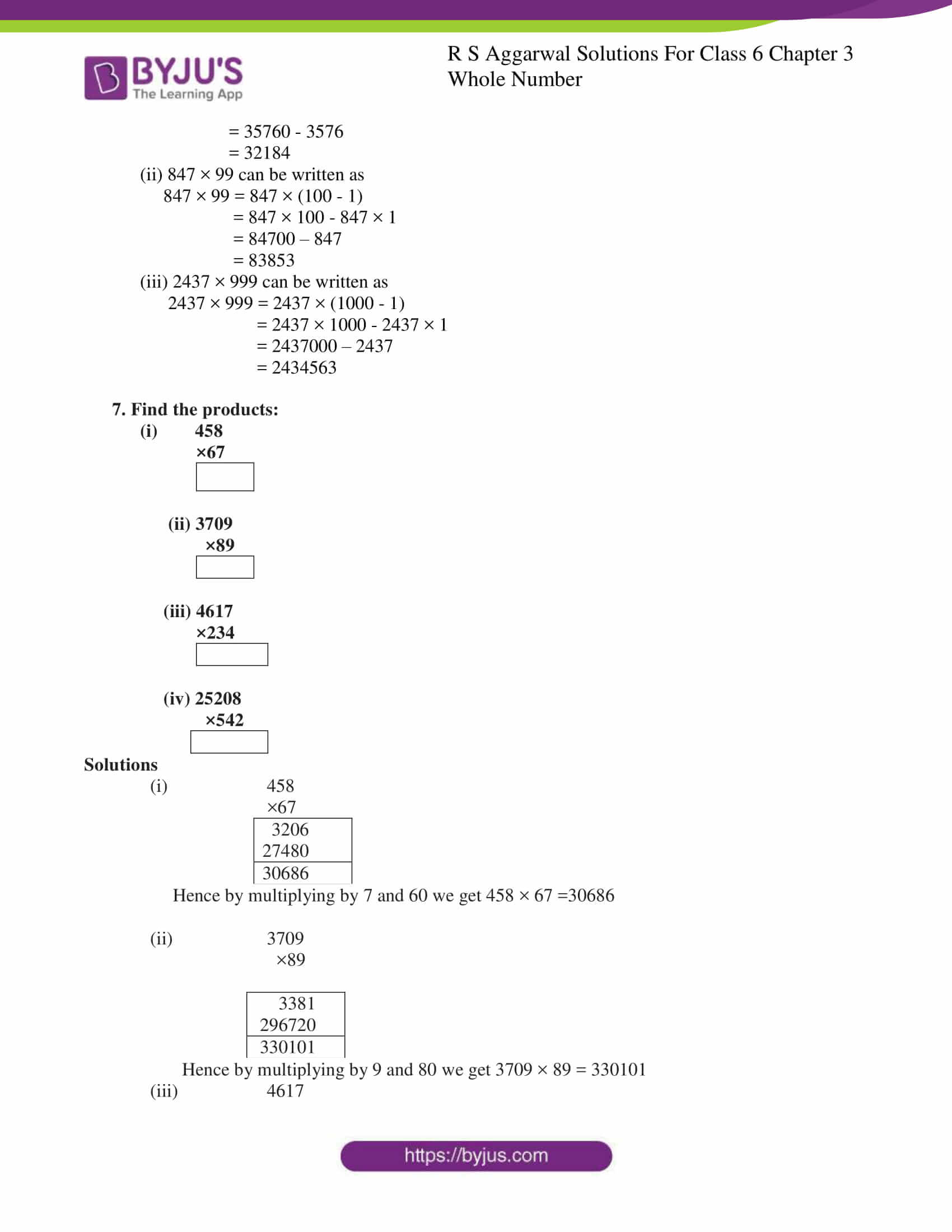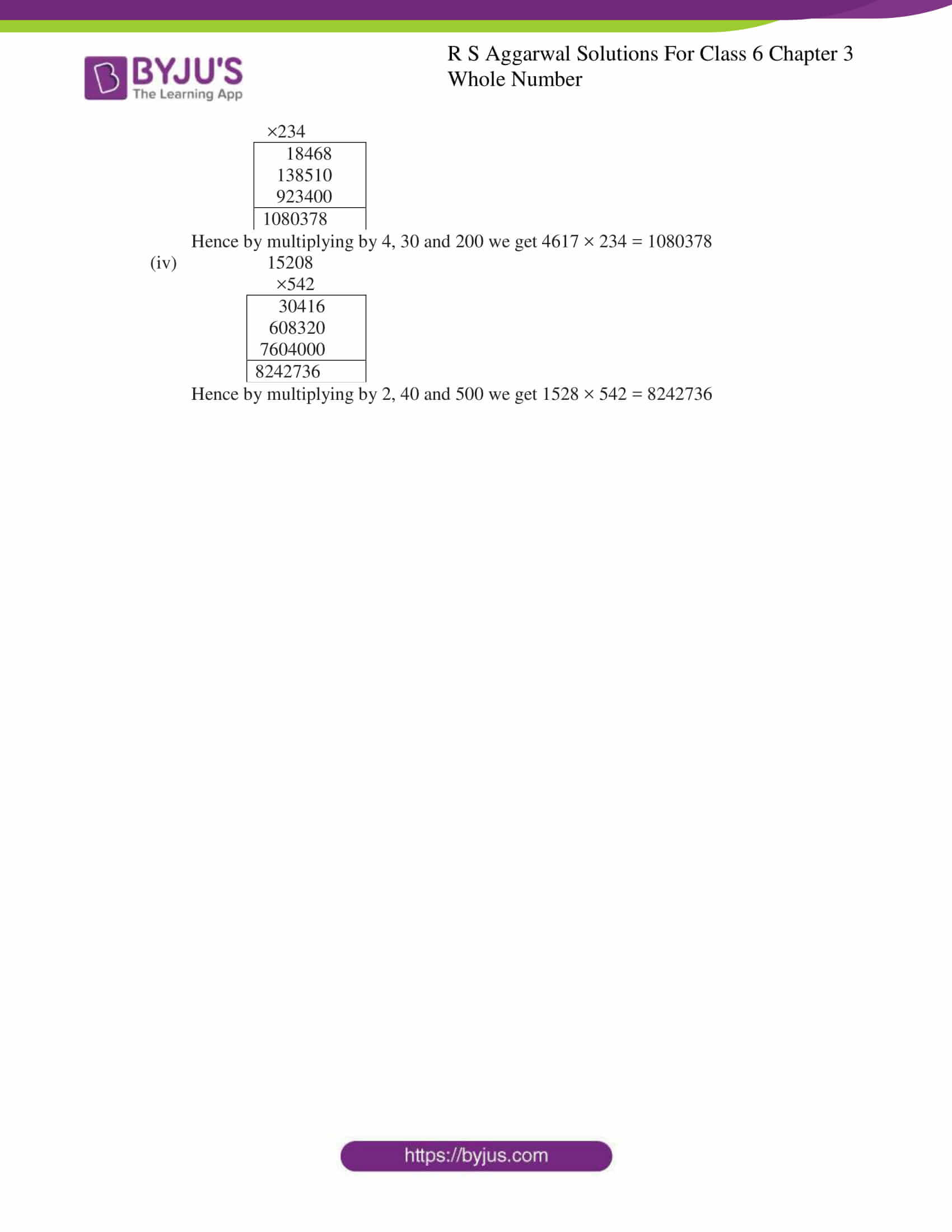### Access answers to Maths RS Aggarwal Solutions for Class 6 Chapter 3 Whole Numbers Exercise 3D

1. Fill in the blanks to make each of the following a true statement:

(i) 246 × 1 = ………

(ii) 1369 × 0 = ……..

(iii) 593 × 188 = 188 × ……..

(iv) 286 × 753 = …….. × 286

(v) 38 × (91 × 37) = ……. × (38 × 37)

(vi) 13 ×100 × …….= 1300000

(vii) 59 × 66 + 59 × 34 = 59 × (……. + …….)

(viii) 68 × 95 = 68 × 100 – 68 ×………

Solution

The true statements are

(i) 246× 1 = 246

(ii) 13690 × 0 = 0

(iii) 593× 188 = 188 × 593

(iv) 286× 753 = 753 × 286

(v) 38× (91 × 37) = (38 × 91) × (38 × 37)

(vi) 13× 100 × 1000 = 1300000

(vii) 59× 66 + 59 × 34 = 59× (66 + 34)

(viii) 68× 95 = 68 × 100 – 68 × 5

2. State the property used in each of the following Statements:

(i) 19 × 17 = 17 × 19

(ii) (16 × 32) is a whole number

(iii) (29 × 36) × 18 = 29 × (36 × 18)

(iv) 1480 × 1 = 1480

(v) 1732 × 0 = 0

(vi) 72 × 98 + 72 × 2 = 72 × (98 + 2)

(vii) 63 × 126 – 63 × 26 = 63 × (126 – 26)

Solutions

(i) 19 × 17 = 17 × 19

⇒ Commutative law of multiplication is used

(ii) (16 × 32) is a whole number

⇒closure property is used

(iii) (29 × 36) × 18 = 29 × (36 × 18)

⇒ Associative of multiplication property is used

(iv) 1480 × 1 = 1480

⇒ Multiplicative identity is used

(v) 1732 ⇒ 0 = 0

⇒ Zero property is used

(vi) 72 × 98 + 72 × 2 = 72 × (98 + 2)

⇒ Distributive law of multiplication over addition is used

(vii) 63 × 126 – 63 × 26 = 63 × (126 – 26)

⇒ Distributive law of multiplication over subtraction is used

3. Find the value of each of the following using various properties:

(i) 647 × 13 + 647 × 7

(ii) 8759 × 94 + 8759 × 6

(iii) 7459 × 999 + 7459

(iv) 9870 × 561 – 9870 × 461

(v) 569 × 17 + 569 × 13 + 569 × 70

(vi) 16825 × 16825 – 16825 × 6825

Solutions

(i) By using distributive property we get

647× 13 + 647 × 7 = 647 × (13 + 7)

= 647 × 20

= 12940

(ii) By using distributive property we get

8759 × 94 + 8759 × 6 = 8759 × (94 + 6)

= 8759 × 100

= 875900

(iii) By using distributive property we get

7459 × 999 + 7459 =7459 × (999 + 1)

= 7459 × 1000

= 7459000

(iv) By using distributive property we get

9870 × 561 – 9870 × 461 = 9870 × (561 – 461)

= 9870 × 100

= 987000

(v) By using distributive property we get

569 × 17 + 569 × 13 + 569 × 70 = 569 × (17 + 13 + 70)

= 569 × 100

= 56900

(vi) By using distributive property we get

16825 × 16825 – 16825 × 6825 = 16825 × (16825 – 6825)

= 16825 × 10000

= 168250000

4. Determine each of the following products by suitable rearrangements:

(i) 2 × 1658 × 50

(ii) 4 × 927 × 25

(iii) 625 × 20 × 8 × 50

(iv) 574 × 625 × 16

(v) 250 × 60 × 50 × 8

(vi) 8 × 125 × 40 × 25

Solutions

(i) It can be written as

2 × 1658 × 50 = (2 × 50) × 1658

= 100 × 1658

= 165800

(ii) It can be written as

4 × 927 × 25 = (4 × 25) × 927

= 100 × 927

= 92700

(iii) It can be written as

625 × 20 × 8 × 50 = (20 × 50) × 8 × 625

= 1000 × 8 × 625

= 8000 × 625

= 5000000

(iv) It can written as

574 × 625 × 16 = 574 × (625 × 16)

= 574 × 10000

= 5740000

(v) It can be written as

250 × 60 × 50 × 8 = (250 × 8) × (60 × 50)

= 2000 × 3000

= 6000000

(vi) It can be written as

8 × 125 × 40 × 25 = (8 × 125) × (40 × 25)

= 1000 × 1000

= 1000000

5. Find each of the following products, using distributive laws:

(i) 740 × 105

(ii) 245 × 1008

(iii) 947 × 96

(iv) 996 × 367

(v) 472 × 1097

(vi) 580 × 64

(vii) 439 × 997

(viii) 1553 × 198

Solutions

(i) Using distributive law of multiplication over addition

We get

740 × 105 = 740 × (100 + 5)

= 740 × 100 + 740 × 5

= 74000 + 3700

= 77700

(ii) Using distributive law of multiplication over addition

We get

245 × 1008 = 245 × (1000 + 8)

= 245 × 1000 + 245 × 8

= 245000 + 1960

= 246960

(iii) Using distributive law of multiplication over subtraction

We get

947 × 96 = 947 × (100 – 4)

= 947 × 100 – 947 × 4

= 94700 – 3788

= 90912

(iv) Using distributive law of multiplication over subtraction

We get

996 × 367 = 367 × (1000 – 4)

= 367 × 1000 – 367 × 4

= 367000 – 1468

= 365532

(v) Using distributive law of multiplication over addition

We get

472 × 1097 = 472 × (1000 + 97)

= 472 × 1000 + 472 × 97

= 472000 + 45784

= 517784

(vi) Using distributive law of multiplication over addition

We get

580 × 64 = 580 × (60 + 4)

= 580 × 60 + 580 × 4

= 34800 + 2320

= 37120

(vii) Using distributive law of multiplication over subtraction

We get

439 × 997 = 439 × (1000 – 3)

= 439 × 1000 – 439 × 3

= 439000 – 1317

= 437683

(viii) Using distributive law of multiplication over addition

We get

1553 × 198 = 1553 × (100 + 98)

= 1553 × 100 + 1553 × 98

= 155300 + 152194

= 307494

6. Find each of the following products, using distributive laws:

(i) 3576 × 9

(ii) 847 × 99

(iii) 2437 × 999

Solutions

Distributive law of multiplication over addition = a (b + c)

= ab + ac

Distributive law of multiplication over subtraction = a (b – c)

= ab – ac

(i) 3576 × 9 can be written as

3576 × 9 = 3576 × (10 – 1)

= 3576 × 10 – 3576 × 1

= 35760 – 3576

= 32184

(ii) 847 × 99 can be written as

847 × 99 = 847 × (100 – 1)

= 847 × 100 – 847 × 1

= 84700 – 847

= 83853

(iii) 2437 × 999 can be written as

2437 × 999 = 2437 × (1000 – 1)

= 2437 × 1000 – 2437 × 1

= 2437000 – 2437

= 2434563

7. Find the products:

(i) 458

×67

(ii) 3709

×89

(iii) 4617

×234

(iv) 25208

×542

Solutions

1. 458

×67

 3206 27480 30686

Hence by multiplying by 7 and 60 we get 458 × 67 =30686

1. 3709

×89

 3381 296720 330101

Hence by multiplying by 9 and 80 we get 3709 × 89 = 330101

1. 4617

×234

 18468 138510 923400 1080378

Hence by multiplying by 4, 30 and 200 we get 4617 × 234 = 1080378

1. 15208

×542

 30416 608320 7604000 8242736

Hence by multiplying by 2, 40 and 500 we get 1528 × 542 = 8242736